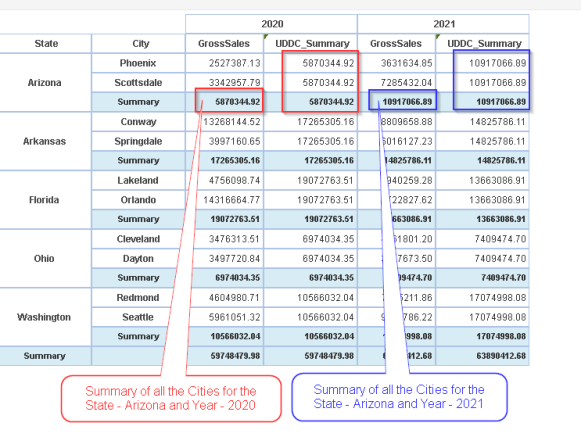· Crosstabs with multiple Row and Column dimensions1) Derive summary on second level (or any levelRow dimension

Description:

Calculate the summary value of second-level (or any level) Row dimension using summary function.

UDDC Expression:

UDDC Expression for calculating Summary:

 summary( rowDimension, ””, Measure )

Example:

UDDC Expression for calculating Summary of second level Row (City) Dimension - UDDC_Summary:

 summary(City, "", GrossSales )

As per the below example,

there are two dimensions kept in the row. They are state (1st ) and city (2nd ).

For the year 2020, the sum of Gross sales of all the cities of Arizona State is 5870344.92. Hence the UDDC_Summary value is 5870344.92

For the year 2021, the sum of Gross sales of all the cities of Arizona State is 10917066.89. Hence the UDDC_Summary value is 10917066.89.2) Derive summary on second level (or any levelColumn dimension

Description:

Calculate the summary value of second-level (or any level) Column dimension using summary function.

UDDC Expression:

UDDC Expression for calculating Summary:

 summary( “”, ColumnDimension, Measure )

Example:

UDDC Expression for calculating Summary of second level Column (Quarter) Dimension - UDDC_Summary:

 summary(“”, Quarter, GrossSales )

As per the below example,

there are two dimensions kept in the row. They are Year (1st ) and Quarter (2nd ).

For the State Arizona , the sum of Gross sales of all the Quarters of Year 2020  is 5870344.92. Hence the UDDC_Summary value is 5870344.92

For the State Arkansas , the sum of Gross sales of all the Quarters of Year 2020  is 17265305.16 Hence the UDDC_Summary value is 17265305.16# Type of Summary Expression Example 1 Derive summary on second level (or any) Row dimension Summary( Row dimension “”, Measure ) Summary( City,“”, Grosssales ) where City is the second row dimension in the crosstab on which the summary will be calculated. Since we are calculating City wise summary here, the column dimension will be empty (“”). The output will be the state wise sum of gross sales of all the cities. 2 Derive summary on second level (or any) Column dimension Summary (“”, Column Dimension,, Measure) Summary("",Quarter,GrossSales) where Quarter is the second column dimension in the crosstab on which the summary will be calculated. Since we are calculating Quarter wise summary here, the row dimension will be empty (“”). The output will be the year wise sum of gross sales of all the quarters.